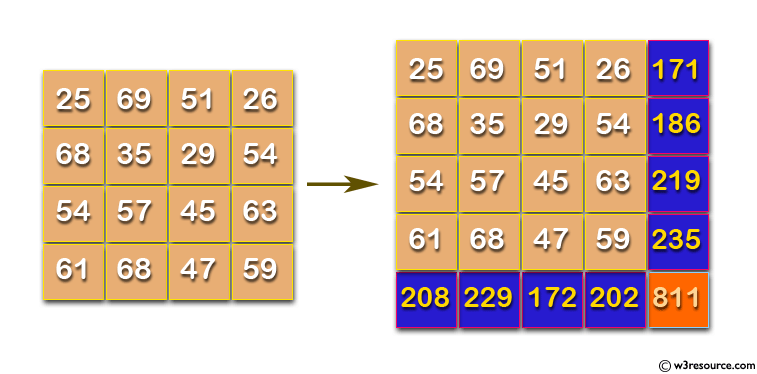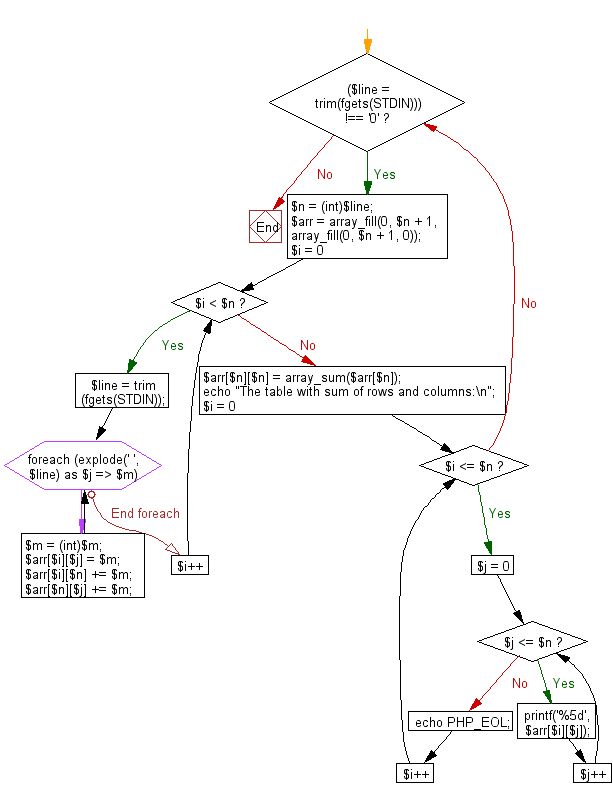﻿ PHP Exercise: Compute the maximum value of the sum of the passing integers - w3resource# PHP Exercises: Compute the maximum value of the sum of the passing integers

## PHP: Exercise-76 with Solution

Write a PHP program which adds up columns and rows of given table as shown in the following figure:

Pictorial Presentation:Input: n (the size of row and column of the given table)
1st row of the table
2nd row of the table
:
:
n th row of the table
The input ends with a line consisting of a single 0.

Sample Output:
The maximum value of the sum of integers passing according to the rule on one line.

Sample Solution: -

PHP Code:

``````<?php
while ((\$line = trim(fgets(STDIN))) !== '0') {
\$n = (int)\$line;
\$arr = array_fill(0, \$n + 1, array_fill(0, \$n + 1, 0));
for (\$i = 0; \$i < \$n; \$i++) {
\$line = trim(fgets(STDIN));
foreach (explode(' ', \$line) as \$j => \$m) {
\$m = (int)\$m;
\$arr[\$i][\$j] = \$m;
\$arr[\$i][\$n] += \$m;
\$arr[\$n][\$j] += \$m;
}
}
\$arr[\$n][\$n] = array_sum(\$arr[\$n]);
echo "The table with sum of rows and columns:\n";

for (\$i = 0; \$i <= \$n; \$i++) {
for (\$j = 0; \$j <= \$n; \$j++) {
printf('%5d', \$arr[\$i][\$j]);
}
echo PHP_EOL;
}
}
?>
```
```

Sample Input:
4
25 69 51 26
68 35 29 54
54 57 45 63
61 68 47 59
0

Sample Output:

```The table with sum of rows and columns:
25   69   51   26  171
68   35   29   54  186
54   57   45   63  219
61   68   47   59  235
208  229  172  202  811```

Flowchart:PHP Code Editor:

Have another way to solve this solution? Contribute your code (and comments) through Disqus.

What is the difficulty level of this exercise?

Test your Programming skills with w3resource's quiz.

﻿

## PHP: Tips of the Day

Why shouldn't I use mysql_* functions in PHP?

The MySQL extension:

• Is not under active development
• Is officially deprecated as of PHP 5.5 (released June 2013).
• Has been removed entirely as of PHP 7.0 (released December 2015)
• This means that as of 31 Dec 2018 it does not exist in any supported version of PHP. If you are using a version of PHP which supports it, you are using a version which doesn't get security problems fixed.
• Lacks an OO interface
• Doesn't support:
• Non-blocking, asynchronous queries
• Prepared statements or parameterized queries
• Stored procedures
• Multiple Statements
• Transactions
• The "new" password authentication method (on by default in MySQL 5.6; required in 5.7)
• Any of the new functionality in MySQL 5.1 or later

Since it is deprecated, using it makes your code less future proof.

Lack of support for prepared statements is particularly important as they provide a clearer, less error-prone method of escaping and quoting external data than manually escaping it with a separate function call.

Ref : https://bit.ly/2BIIsF0